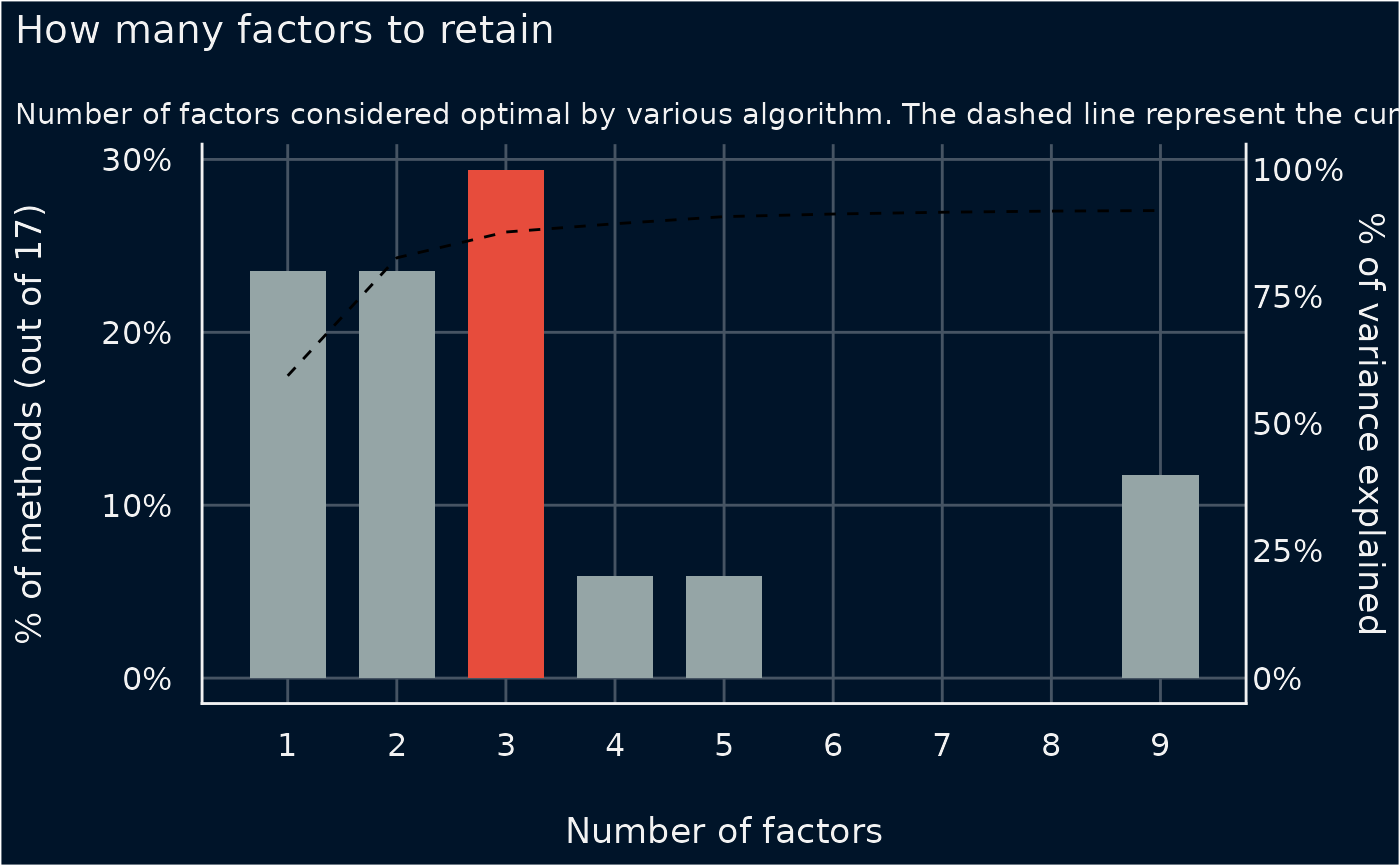The plot() method for the parameters::n_factors() and parameters::n_clusters()

## Usage

# S3 method for see_n_factors
plot(x, data = NULL, type = c("bar", "line", "area"), size = 1, ...)

## Arguments

x

An object.

data

The original data used to create this object. Can be a statistical model.

type

Character vector, indicating the type of plot.

size

Depending on type, a numeric value specifying size of bars, lines, or segments.

...

Arguments passed to or from other methods.

## Value

A ggplot2-object.

## Examples

if (require("parameters") && require("nFactors")) {
data(mtcars)
result <- n_factors(mtcars, type = "PCA")
result
plot(result, type = "line")
}